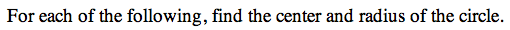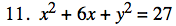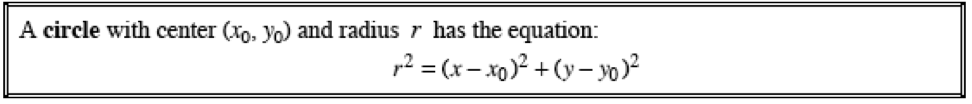13C-11.

### Missing problem for Book PCT => Chapter Ch13 => Lesson 13.1.1 => Problem 13C-11

Created from orphaned homework help problemYou'll need to complete the square to get the equation in Standard Form.

(x² + 6x + 9) + y² = 27 + 9